Courses

# Finding areas using integration, Business Mathematics & Statistics B Com Notes | EduRev

## B Com : Finding areas using integration, Business Mathematics & Statistics B Com Notes | EduRev

The document Finding areas using integration, Business Mathematics & Statistics B Com Notes | EduRev is a part of the B Com Course Business Mathematics and Statistics.
All you need of B Com at this link: B Com

## Area Under a Curve

Calculating the area under a curve.

Definite Integrals

So far when integrating, there has always been a constant term left. For this reason, such integrals are known as indefinite integrals. With definite integrals, we integrate a function between 2 points, and so we can find the precise value of the integral and there is no need for any unknown constant terms [the constant cancels out].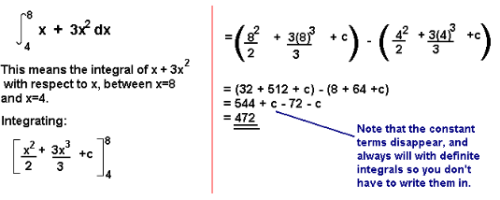The Area Under a Curve

The area under a curve between two points can be found by doing a definite integral between the two points.

To find the area under the curve y = f(x) between x = a and x = b, integrate y = f(x) between the limits of a and b.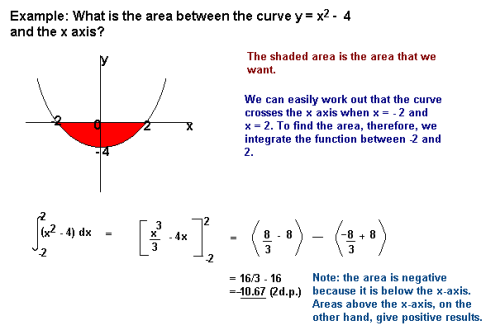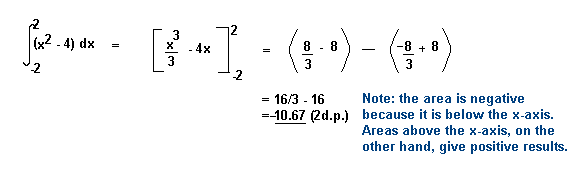Areas under the x-axis will come out negative and areas above the x-axis will be positive. This means that you have to be careful when finding an area which is partly above and partly below the x-axis.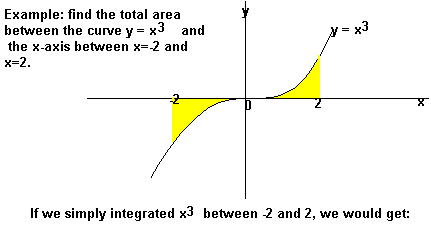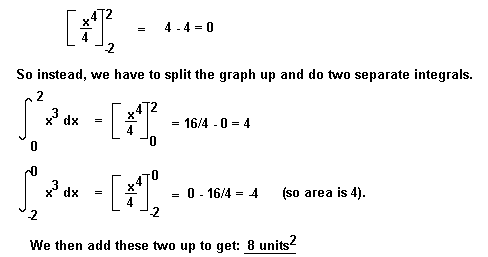You may also be asked to find the area between the curve and the y-axis. To do this, integrate with respect to y.

Example

Find the area bounded by the lines y = 0, y = 1 and y = x2.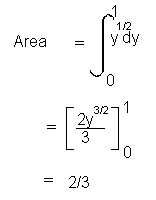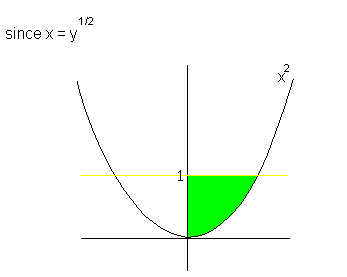Offer running on EduRev: Apply code STAYHOME200 to get INR 200 off on our premium plan EduRev Infinity!

122 videos|142 docs

,

,

,

,

,

,

,

,

,

,

,

,

,

,

,

,

,

,

,

,

,

,

,

,

;